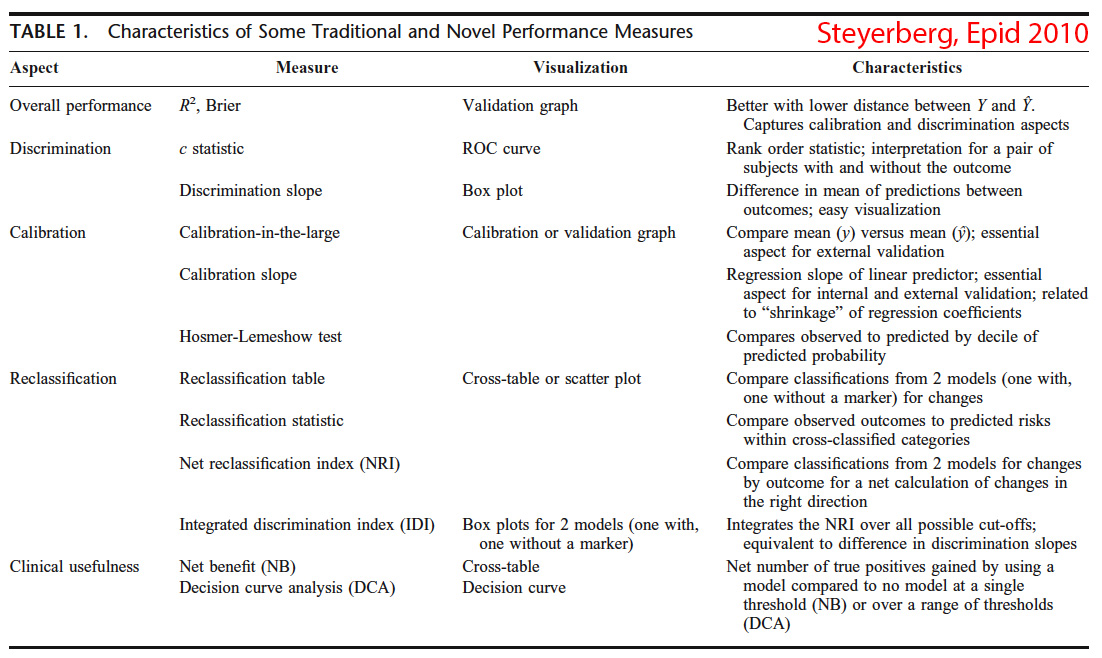# Assessing a model: Deviance, Calibration, -2 log likelihood

The performance of a logistic regression model can be described along multiple dimensions:

• overall model fit (deviance, Brier score, explained $$R^2$$)
• discrimination (C index)
• calibration (global: calibration graph; intercept and slope of calibration curve, shrinkage)
• clinical usefulness: net number of true positives gained by using the model (vs. no model) at a single threshold (NB=net benefit) or over a range of thresholds (DCA= decision curve analysis)Steyerberg, Epidemiology 2010; 21:128

## Goodness-of-fit

### Deviance

In logistic regression, there is no true $$R^2$$ statistic. Instead, we have deviance, which is analogous to the sum of residual squares in OLS restriction. Deviance is referred to as $$-2\ log\ likelihood$$ or $$-2LL$$ or $$D$$. A smaller deviance is better.
The deviance test is an example of a logarithmic scoring rule (Nagelkerke’s $$R^2$$ a.k.a. the explained variation, is another), while the Brier score is a quadratic scoring rule. The logarithmic scoring rule is a local strictly proper scoring rule. This is also the negative of surprise, which is commonly used as a scoring criterion in Bayesian Inference; the goal is to minimize expected surprise. This scoring rule has strong foundations in information theory.
If one treats the truth or falsity of the prediction as a variable x with value 1 or 0 respectively, and the expressed probability as p, then one can write the logarithmic scoring rule as $x*ln(p) + (1-x)*ln(1-p)$ A prediction of 80% that correctly proved true would receive a score of ln(0.8) = -0.22. This same prediction also assigns 20% likelihood to the opposite case, and so if the prediction proves false, it would receive a score based on the 20%: ln(0.2) = -1.6. The goal of a forecaster is to maximize the score and for the score to be as large as possible, and -0.22 is indeed larger than -1.6.

### The Likelihood Ratio Statistic

It is a goodness of fit, showing how many times more likely the date are under the model compared to a null model. Due to its distribution, this is sometimes referred to as $$Likelihood\ ratio\ \chi^2$$. $D=-2*ln\frac{Likelihood_{null}}{likelihood_{model}}\ = 2*[ln(L_{model})-ln (L_{null})]$ Of note $$-2LL_{null}$$ measures the deviance with the intercept only. The model with more parameters (here model) will always fit at least as well, i.e., have a greater or equal log-likelihood, as the model with less parameters (here null).
The likelihood-ratio test requires nested models, i.e. models in which the more complex one can be transformed into the simpler model by imposing a set of constraints on the parameters (e.g. set them to zero).

### Brier score

It is a quadratic scoring rule (vs. a log scoring rule e.g. $$-2LL$$) that measures the accuracy of probabilistic predictions of a set of mutually exclusive discrete outcomes. Is the mean squared difference between predicted and the actual outcome; in other words the mean squared error. Ranges from 0 (for the perfect model) to 0.25 (for the noninformative model); therefore lower is better. It is possible to use a scaled Brier score which is very similar to Pearson’s $$R^2$$ (Steyerberg 2010)

## Discrimination

### C index

The C index (probability of concordance between predicted probability and response) is a unitless measure of the fitted model’s predictive discrimination. Measures the rank correlation between predicted prob of response and actual response. It is identical to the AUC-ROC and it is related to Somers’ $$D_{xy}$$ rank correlation between predicted probabilities and observed responses (diff between concordance and discordance probabilities). $D_{xy} = 2*(c-0.5)$ When $$D_{xy}$$ is 0 the model is random.

## Calibration

Calibration refers to the observed agreement between predictions and observed outcomes. Can be represent graphically and the curve’s intercept describes the “calibration-at-large” i.e. the extent to which the predictions are systematically too low or too high. At the time of validation, intercept problems become apparent as are issues of overfitting, meaning that the slope of the curve will be <1. The overfitting should be anticipated and corrected in part by shrinkage.

The significance of the individual predictors is described by the Wald $$\chi^2$$ test or the LR test (above). Occasionally by the Lagrange multiplier test (score test) can be used (Newsom notes)